# Inverted MMI

19 December 2019

### Create a MMI using custom interconnects and polygon manipulation

This example demonstrates how to create a building block with optional layer inversion. Along the way the example shows Nazca’s interconnect features and polygon operations. It shows some more “advanced” ways to use Nazca. The code snippet below shows what is needed by the end-user/design to create the MMI layout displayed on the right (after defining the building block and interconnects, as shown further down).

```ic.strt(length=30).put(0)
m = mmi(length=50).put()
ic.bend(angle=-30).put()
ic.bend(angle=30).put(m.pin['b1'])

# inverted version:
ici.strt(length=30).put(0, -50)
mi = mmi(length=50, inverse=True).put()
ici.bend(angle=-30).put()
ici.bend(angle=30).put(mi.pin['b1'])

nd.export_gds()```

The code below provides all the parts needed from scratch, e.g. when you create a library or process design kit. It creates the mask layers, an inverted and non-inverted xsection, a custom tapered-sinebend interconnect and the definition of the MMI building block.

The MMI building block consists of two layers, the waveguide and a trench overlay layer. The inverted MMI subtracts the waveguide from the trench to obtain a “trench-only” mask layer. Two auxiliary functions are demonstrated, i.e. “merge_polygon” and “remove_polygons”. The first merges polygons in the relevant layers of the MMI cell to be able to subtract layers for the inversion, and the second one (optionally) deletes layers that were used to generate the inverted MMI. The polygon operations make use of the Nazca cell_iter that allows for extremely versatile “cell-surgery”.

Note that the Nazca polygon operations are universal and not particular for this MMI, hence, they can be reused. Also note that there may be some limitations in the pyclipper module that does the actual merging and/or diffing , so not all building block geometries may work out of the box.

```# example created by Bright Photonics
from math import sin, pi
from collections import defaultdict
import nazca as nd

# create layers:

# create xs + interconnect

# create inverted xs + interconnect
nd.add_layer2xsection(xsection='xs1i', layer='lay3', leftedge=(0.5, 0), rightedge=(0.5, 5))
nd.add_layer2xsection(xsection='xs1i', layer='lay3', leftedge=(-0.5, 0), rightedge=(-0.5, -5))

# create a custom tapered raised sinebend and add it as an interconnect to 'ic':
def x(t, **kwargs): return 20*t
def y(t, offset, **kwargs): return offset * (sin(2*pi*t) - 2*pi*t)
def w(t, width1, width2, **kwargs): return width1 + (width2-width1)*t
ic.tsinebend = ic.Tp_viper(x=x, y=y, w=w, offset=1.0)
# Adding the viper as interconnect is nazca.0.5.9 (ic.Tp_viper)
# Use nd.Tp_viper otherwise and provide explicit width1 and width2 values

# define auxiliary cell/polygon manipulation functions:
def merge_polygons(cell, layers):
"""Merge all polygons per layer after flattening <cell>.

Cell <cell> itself will not be affected. Note that a merged polygons may
still consist of multiple polygons (islands).

Args:
cell (Cell): cell to process
layers (list of str): names of layers to merge polygons in

Returns:
dict: {layer_name: merged_polygon}
"""
pgons = defaultdict(list)
for params in nd.cell_iter(cell, flat=True):
for pgon, xy, bbox in params.iters['polygon']:
for lay in layers:
if pgon.layer == lay:
pgons[lay].append(xy)
for lay in layers:
pgons[lay] = nd.clipper.merge_polygons(pgons[lay])
return pgons

def remove_polygons(cell, layers):
"""Remove all polygons in <layers> from <cell>.

Args:
cell (Cell): cell to process
layers (list of str): names of layers to delete polygons from

Returns:
None
"""
for params in nd.cell_iter(cell):
if params.cell_start:
pgons = []
for pgon in params.cell.polygons:
if pgon.layer not in layers:
pgons.append(pgon)
params.cell.polygons = pgons
return None

# define the actual MMI building block:
def mmi(length=30, inverse=False):
"""Create a custom 1x2 MMI.

Args:
length (float): length of the mmi body
inverse (bool): if True create trench only. Default=False

Returns:
Cell: 1x2 MMI element
"""
with nd.Cell(name=f'mmi_{length}_{inverse}') as C:
C.autobbox = True
ic.strt(length=length, width=20, arrow=False).put(0)
ic.tsinebend(width1=6.0, offset=1.0, arrow=False).put(length, -5)
o1 = ic.strt(length=5.0, arrow=False).put()
ic.tsinebend(width1=6.0, offset=-1.0, arrow=False).put(length, 5)
o2 = ic.strt(length=5.0, arrow=False).put()
# example of connecting the input taper "backwards"
t = ic.ptaper(length=10.0, width2=8.0, arrow=False).put('b0', 0, 0, 180)
i1 = ic.strt(length=5.0, arrow=False).put('b0', t.pin['a0'])

clad = [(0, -20), (-15, 0), (0, 20), (length+5, 20), (length+25, 17),
(length+25, -17), (length+5, -20)]

nd.Pin('a0', pin=i1.pin['a0']).put()
nd.Pin('b0', pin=o1.pin['b0']).put()
nd.Pin('b1', pin=o2.pin['b0']).put()
nd.put_stub() # show the pins in the layout

if inverse:
pgons = merge_polygons(cell=C, layers=['lay1', 'lay2'])
remove_polygons(cell=C, layers=['lay1', 'lay2']) # optional
pdiff = nd.clipper.diff_polygons(pgons['lay2'], pgons['lay1'])
for pol in pdiff:
nd.Polygon(points=pol, layer='lay3').put(0)
return C

# Create a design using the non-inverted and inverted implementation:
ic.strt(length=30).put(0)
m = mmi(length=50).put()
ic.bend(angle=-30).put()
ic.bend(angle=30).put(m.pin['b1'])

ici.strt(length=30).put(0, -50)
mi = mmi(length=50, inverse=True).put()
ici.bend(angle=-30).put()
ici.bend(angle=30).put(mi.pin['b1'])

nd.export_gds()```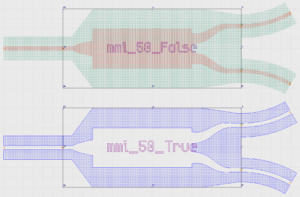## Related Tutorials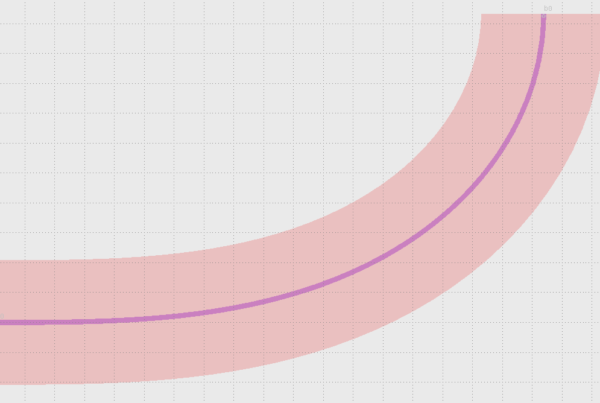In this example we show how to use Euler bends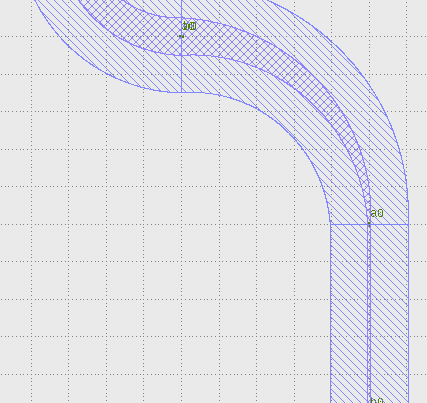In this example we show how to create a free form parametric curve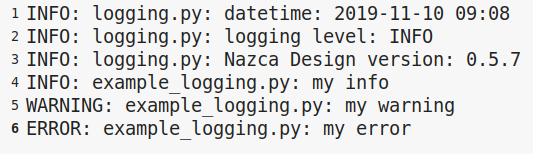In this example we show how to log your layout.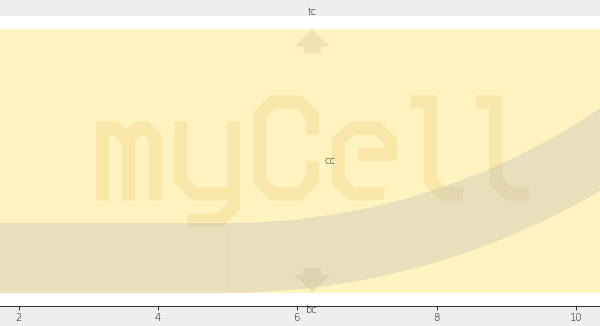In this example we show how to create xsections and layers